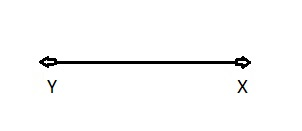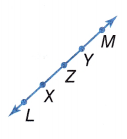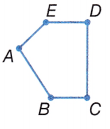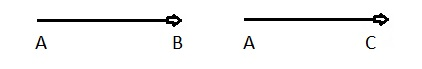# McGraw Hill Math Grade 8 Lesson 18.2 Answer Key Line Segments and Rays

Practice the questions of McGraw Hill Math Grade 8 Answer Key PDF Lesson 18.2 Line Segments and Rays to secure good marks & knowledge in the exams.

## McGraw-Hill Math Grade 8 Answer Key Lesson 18.2 Line Segments and Rays

Exercises

SOLVE

Question 1.
Draw a diagram that illustrates a ray $$\overrightarrow{Y X}$$.Explanation:
Drawn a diagram that illustrated a ray $$\overrightarrow{Y X}$$ as shown above.

Question 2.
Identify two rays and two line segments in the figure.Two rays: $$\overrightarrow{XL}$$, $$\overrightarrow{YM}[/latex,Two line segments: LX,YM Explanation: Identified two rays [latex]\overrightarrow{XL}$$, $$\overrightarrow{YM}$$ as rays means a part of a line that has one endpoint and goes on infinitely in only one direction. Two line segments LX, YM as in geometry, a line segment is bounded by two distinct points on a line also a line segment is part of the line that connects two points.

Question 3.
Identify three line segments in the diagram.Three line segments: AB, BC, CD,

Explanation:
The three line segments in the given diagram above are AB, BC, CD.

Question 4.
Draw two rays $$\overrightarrow{A B}$$ and $$\overrightarrow{A C}$$.Explanation:
Drawn two rays $$\overrightarrow{A B}$$ and $$\overrightarrow{A C}$$ as shown above.

Question 5.
Identify the two line segments.